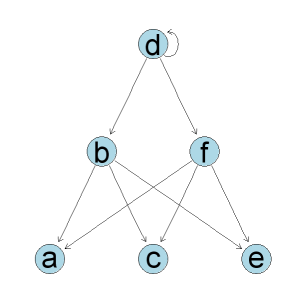# Drawing network graphs (nodes and edges) with R/BioConductor

How do you draw network graphs in R? - the mathematical type of graph made up of nodes and edges that is.

Several people recommended an R/BioConductor package called Rgraphviz, which is an interface to Graphviz - Graph Visualization Software. You'll need to install R, BioConductor and Graphviz first, and then install the Rgraphviz package and its dependancies from within R as follows:

```source("http://www.bioconductor.org/biocLite.R")
biocLite("Ruuid")
biocLite("Rgraphviz")
```

So, documentation:

If you are more interested in the Graphviz and its DOT file format you could try:

Here is a simple example converting an adjacency matrix into a graphAM object and plotting it. The matrix elements are taken to be the edge weights (zero being the absence of an edge), with the row and column names asssumed to be the nodes.

```> library(Rgraphviz)
...
> test.matrix<-matrix(rep(c(0,1,0,0), 9), ncol=6, nrow=6)
> rownames(test.matrix)<-c("a", "b", "c", "d", "e", "f")
> colnames(test.matrix)<-c("a", "b", "c", "d", "e", "f")
> test.matrix
a b c d e f
a 0 0 0 0 0 0
b 1 0 1 0 1 0
c 0 0 0 0 0 0
d 0 1 0 1 0 1
e 0 0 0 0 0 0
f 1 0 1 0 1 0
> am.graph
A graphAM graph with directed edges
Number of Nodes = 6
Number of Edges = 9
> plot(am.graph, attrs = list(node = list(fillcolor = "lightblue"),
edge = list(arrowsize=0.5)))```

BioConductor also has a graphNEL object that uses Nodes and Edge-List internally.

You can also use the as command to convert matrices into graphs, but using new is recommended as it gives you more control. For this simple example it makes no difference:

```> am.graph <- as(test.matrix, Class="graphAM")
> am.graph
A graphAM graph with directed edges
Number of Nodes = 6
Number of Edges = 9

> nel.graph <-as(test.matrix, Class="graphNEL")
> nel.graph
A graphNEL graph with directed edges
Number of Nodes = 6
Number of Edges = 9 ```

The as command can also convert between the NEL and AM graph objects:

```> as(am.graph, Class="graphNEL")
A graphNEL graph with directed edges
Number of Nodes = 6
Number of Edges = 9

> as(nel.graph, Class="graphAM")
A graphAM graph with directed edges
Number of Nodes = 6
Number of Edges = 9```